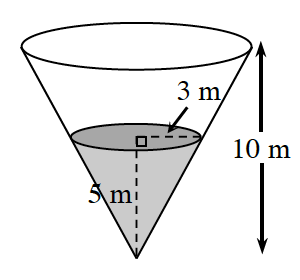### Home > PC3 > Chapter 7 > Lesson 7.2.4 > Problem7-120

7-120.

The amount of water evaporating from a conical container varies directly with the exposed surface area (circular region) of the water in the cone. The height of the cone is $10$ meters. When the height of the water is $5$ meters, the radius of the exposed circular region is $3$ meters. At this point, water is evaporating at a rate of $0.2$ liters per hour.

1. How fast is the water evaporating when the container is full?

Determine the radius when the cone is filled.

$\frac{3}{5}=\frac{r}{10}$

Set up and solve a proportion relating the area with the rate of evaporation.

$\frac{9\pi}{0.2}=\frac{36\pi }{x}$

2. How fast is the water evaporating when it is half full? (Reminder $V=\frac{1}{3}\pi r^2h$.)

When the cone is full, it has $120\pi$ m3 of water in it.
What is the volume of water in the cone when it is half full?

Calculate $r$ when the cone is half full. Then set up a proportion with area and evaporation rate similar to the one in part (a).